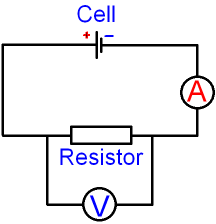gcsescience.com                                       10                                       gcsescience.com

Electricity

What is a Resistor?

A resistor is a component that decreases the current in
a circuit. A resistor transfers electrical energy into
heat energy. See the theory of the resistance of wires.

How is Resistance Calculated?

We can calculate resistance using the equation

voltage = current x resistance     V = I x R.

Firstly, we need to measure the current flowing
through the resistor and the voltage across the resistor.The circuit diagram above shows
where to place an ammeter and a voltmeter.

The equation
voltage = current x resistance   V = I x R

can be rearranged to give
r
esistance = voltage ÷ current    R = V ÷ I

If the ammeter reads 2 A, and the voltmeter reads 6 V,

then   R = V ÷ I
= 6 ÷ 2

= 3 ohms.

The same method can be used to
calculate the resistance of any component.

gcsescience.com        Physics Quiz        Index        Electricity Quiz        gcsescience.com